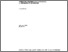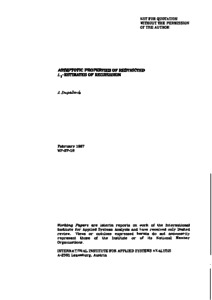Asymptotic Properties of Restricted L1-Estimates of Regression

Dupacova, J. (1987). Asymptotic Properties of Restricted L1-Estimates of Regression. IIASA Working Paper. IIASA, Laxenburg, Austria: WP-87-018Preview
Text
WP-87-018.pdf

Abstract

Asymptotic properties of L_{1}-estimates in linear regression have been studied by many authors, see e.g. Bassett and Koenker (1978), Bloomfield and Steiger (1983). It is the lack of smoothness which does not allow to we the known results on asymptotic behavior of M-estimates directly (Huber (1967)). The additional lack of a convexity in the nonlinear regression case increases the complexity of the problem even under assumption that the true parameter values belong to the interior of the given parameter set; for a consistency result in this case see e.g. Oberhofer (1982).

We shall use the technique developed in Dupacova and Wets (1986, 1987) to get asymptotic properties of the L_{1}-estimates of regression coefficients which are assumed to belong to an a priori given closed convex set given, e.g., by constraints of general equality and inequality form. The method uses, i.a., tools of nondifferentiable calculus and epi-convergence and it can be applied to other classes of L_{1}-estimates as well.

Item Type: Monograph (IIASA Working Paper) Adaption and Optimization (ADO) IIASA Import 15 Jan 2016 01:58 27 Aug 2021 17:13 http://pure.iiasa.ac.at/3033View Item